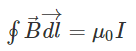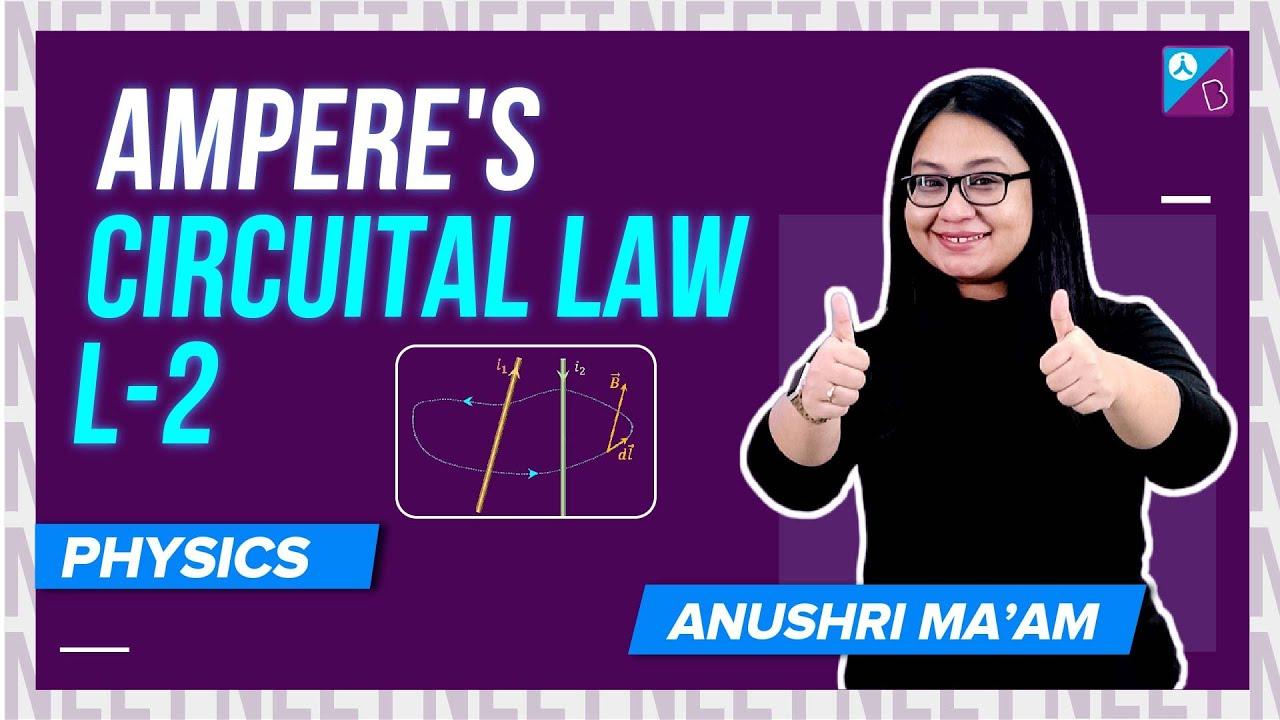Win up to 100% scholarship on Aakash BYJU'S JEE/NEET courses with ABNAT Win up to 100% scholarship on Aakash BYJU'S JEE/NEET courses with ABNAT

# Amperes Law MCQs

Ampere’s Law can be stated as:

“The magnetic field created by an electric current is proportional to the size of that electric current with a constant of proportionality equal to the permeability of free space.”• B is the magnetic field
• L is the infinitesimal length
• I is the current flowing through the closed-loop
• μ is the permeability

Q1: If a coil carrying current is placed in a uniform magnetic field, then

1. emf is produced
2. Torque is produced
3. Force is produced
4. Torque and force is produced

Q2: A coil of diameter 0.2 cm is formed by a 6.28 m long wire and a current of 1 amp is passed in it. The magnetic induction at its centre will be

1. 6.28 x 10-5 T
2. 7.25 x 10-5 T
3. 6.28 x 10-2 T
4. 6.28 T

Answer: (a) 6.28 x 10-5 T

Q3: A long wire carrying a steady current is bent into a circular loop of one turn. The magnetic field at the centre of the loop is B. It is then bent into a circular coil of n turns. The magnetic field at the centre of this coil of n turns will be

1. 2Bn2
2. n2B
3. n3B
4. nB

Q4: A long straight wire of radius a carries a steady current I. The current is uniformly distributed over its cross-section. The ratio of the magnetic fields B and B’, at radial distances a/2 and 2a respectively from the axis of the wire is:

1. ¼
2. ½
3. 1
4. 4

Q5: Compute the magnetic field of a long straight wire that has a circular loop with a radius of 0.05 m. 2 amp is the reading of the current flowing through this closed loop.

1. 8 x 10-6 T
2. 8 T
3. 8 x 10-7 T
4. 7 x 10-7 T

Answer: (a) 8 x 10-6 T

Q6: A wire of length L carrying current I is bent into a circle of one turn. The field at the centre of the coil is B1. A similar wire of length L carrying current I is bent into a square of one turn. The field at its centre is B2. Then

1. B1=B2
2. B1＞B2
3. B2＞B1
4. B1/B2 =2

Q7: Two long straight parallel wires carry currents I1 and I2, respectively, in the same direction (as shown). The distance between the wires is R. The magnetic field at the centre of the two wires will

1. μ0(I1-I2)/πR into the plane of the paper (if I1＞I2)
2. μ0(I2-I1)/πR out the plane of the paper (if I2＞I1)
3. μ0(I1-I2)/πR2 out the plane of the paper (if I2＞I1)
4. μ0(I2-I1)/πR2, into the plane of the paper (if I1＞I2)

Answer: (a) μ0(I1-I2)/πR into the plane of the paper (if I1＞I2)

Q8: Two parallel long wires carry currents i1 and i2 with i1 ＞i2. When the current is in the same direction, the magnetic field at a point midway between the wire is 20μT If the direction of i1 is reversed the field becomes 30μT. The ratio of i1/i2 is

1. 4
2. 3
3. 2
4. 1

Q9: A large metal sheet carries an electric current along its surface. Current per unit length is λ. Magnetic field near the metal sheet is

1. (½)μ0λ
2. μ0λ/2π
3. Μ0λ
4. μ0/λ2π

Q10: Charged particle ‘q’ moving through a magnetic field with velocity V will have zero magnetic force when the angle is

1. 0
2. 90
3. 180
4. Both a and b

Answer: (d) Both a and b

Related NEET Physics articles: